# Luna Tech

Tutorials For Dummies.

# 0. 前言

Reference: 8.18

You might conclude from this that there is some underlying structure to the web that clusters together web sites that are similar on some level.

One graph algorithm that can help find clusters of highly interconnected vertices in a graph is called the strongly connected components algorithm (SCC).

# 1. Strongly Connected Components (SCC)

## 定义

strongly connected component - C

C 属于一个 Graph - G

C 需要满足这个条件：在 C 里面的每一对 node（如 v, w），都有 v 到 w 的 edge 以及 w 到 v 的 edge（也就是双向关联）。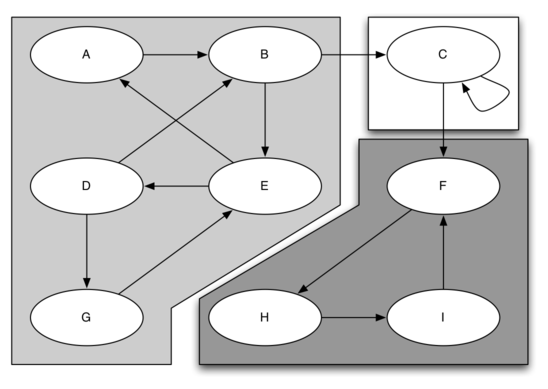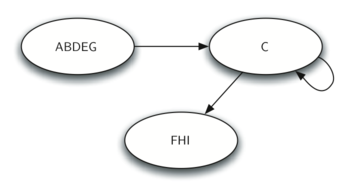# 2. 概念：Transpose Graph

The transposition of a graph 𝐺 is defined as the graph $𝐺^𝑇$ where all the edges in the graph have been reversed.

## 转置前：G

SCC: ABD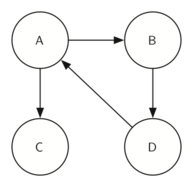## 转置后 $G^T$

SCC: ABD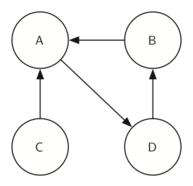# 3. SCC 步骤

SCC 算法用来计算 graph 里面的 SCC，它基于我们之前讲过的 DFS。

## 步骤

1. 在 G 里面调用 dfs function，计算每个 node/vertex 的 finishing time；
2. 计算 $G^T$
3. 在 $G^T$ 里面调用 dfs function，但是 DFS 最主要的那个 loop 是以 desceding order of finish time 来遍历 node/vertex 的；
4. Step 3 的结果是一个 forest，这个 forest 里面的每棵树都是 SCC，我们最终输出在 forest 里面的每棵树的每个 node 的 id（一个 list），用来标识 SCC。

## 图解步骤

### 0. Graph### 1. 计算 G 的 start & finish time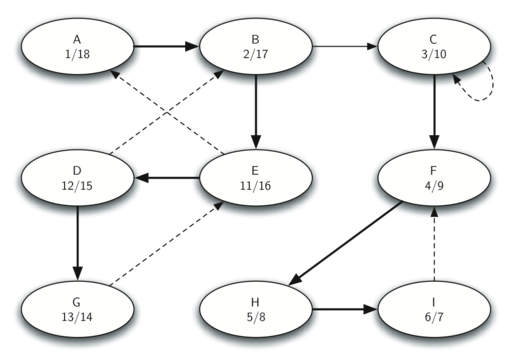### 2. 计算 $G^T$ 的 start & finish time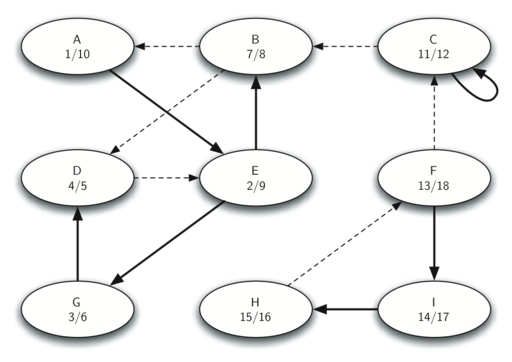### 3. Forest of SCC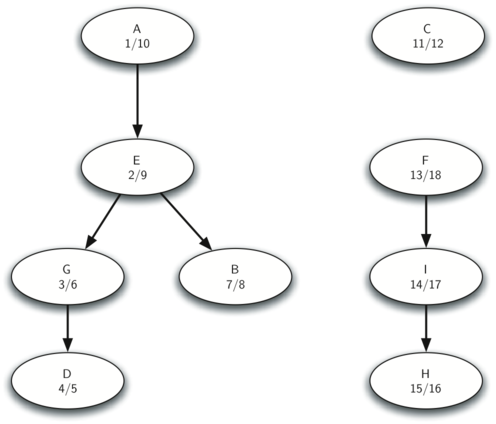# 思考

## 为什么要转置？

https://en.wikipedia.org/wiki/Transpose_graph

Although there is little difference mathematically between a graph and its transpose, the difference may be larger in computer science, depending on how a given graph is represented. For instance, for the web graph, it is easy to determine the outgoing links of a vertex, but hard to determine the incoming links, while in the reversal of this graph the opposite is true. In graph algorithms, therefore, it may sometimes be useful to construct the reversal of a graph, in order to put the graph into a form which is more suitable for the operations being performed on it. An example of this is Kosaraju’s algorithm for strongly connected components, which applies depth first search twice, once to the given graph and a second time to its reversal.